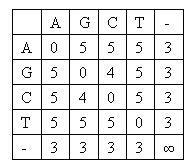Pku2520 DNA Sequence Alignment

题目描述

Gnaileux Iew is attracted in Bioinformatics recently. He reads papers day and night and devotes all his mind in studying. Today he is going to review the basic problem in Bioinformatics: DNA sequence alignment. His purpose is to find a simple and effective algorithm that performs global alignment with two highly similar DNA sequences.

A DNA sequence is presented as a sequence of characters, which may be 'A', 'G', 'C' or 'T'. To align two DNA sequences, some gaps may be inserted to sequences so that two sequences have the same length. And then it is counted up for every pair of matched characters by a score matrix. Gnaileux Iew uses a minimal-score matrix hence the total score of alignment should be minimized. Following is the score matrix Gnaileux Iew uses:For example, an alignment for DNA sequences "AAGACG" and "CAGAGCTC" may be:
-AAGA-C-G
CA-GAGCTC
The total score is 3+0+3+0+0+3+0+3+4=16.

Gnaileux Iew is only interested in aligning highly similar sequences. Strictly speaking, |LCS(A,B)| * 2 / (|A |+ |B|) >= 90%, where A and B are the sequences to align, and LCS(A,B) is the longest common subsequence of A and B.

输入格式

contains two lines, which are the two DNA sequences to align. DNA sequences contain only characters 'A', 'G', 'C' and 'T'. The length of each sequence is not greater than 50000.

You can assume that all the input cases are highly similar sequences.

输出格式

For each test case print the minimal total score of alignment in one line.

样例输入

AGTGCTGAAAGTTGCGCCAGTGAC
AGTGCTGAAGTTCGCCAGTTGACG

12